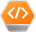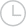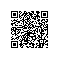# 求Golang精编选择题-问答-阿里云开发者社区-阿里云

## 求Golang精编选择题剑曼红尘2020-03-07 13:41:21880

Golang精编选择题，搞定golang面试

Go取消 提交回答

• 剑曼红尘2020-03-09 10:46:25

1.   【初级】下面属于关键字的是（） A. func B. def C. struct D. class

参考答案：AC

2.   【初级】定义一个包内全局字符串变量，下面语法正确的是（） A. var str string B. str := "" C. str = "" D. var str = ""

3.   【初级】通过指针变量 p 访问其成员变量 name，下面语法正确的是（） A. p.name B. (*p).name C. (&p).name D. p->name

参考答案：AB

4.   【初级】关于接口和类的说法，下面说法正确的是（） A. 一个类只需要实现了接口要求的所有函数，我们就说这个类实现了该接口 B. 实现类的时候，只需要关心自己应该提供哪些方法，不用再纠结接口需要拆得多细才合理 C. 类实现接口时，需要导入接口所在的包 D. 接口由使用方按自身需求来定义，使用方无需关心是否有其他模块定义过类似的接口

参考答案：ABD

5.   【初级】关于字符串连接，下面语法正确的是（） A. str := ‘abc’ + ‘123’ B. str := "abc" + "123" C. str ：= '123' + "abc" D. fmt.Sprintf("abc%d", 123)

参考答案：BD

6.   【初级】关于协程，下面说法正确是（） A. 协程和线程都可以实现程序的并发执行 B. 线程比协程更轻量级 C. 协程不存在死锁问题 D. 通过channel来进行协程间的通信

7.   【中级】关于init函数，下面说法正确的是（） A. 一个包中，可以包含多个init函数 B. 程序编译时，先执行导入包的init函数，再执行本包内的init函数 C. main包中，不能有init函数 D. init函数可以被其他函数调用

参考答案：AB

8.   【初级】关于循环语句，下面说法正确的有（） A. 循环语句既支持for关键字，也支持while和do-while B. 关键字for的基本使用方法与C/C++中没有任何差异 C. for循环支持continue和break来控制循环，但是它提供了一个更高级的break，可以选择中断哪一个循环 D. for循环不支持以逗号为间隔的多个赋值语句，必须使用平行赋值的方式来初始化多个变量  参考答案：CD

9.   【中级】对于函数定义：

1. func add(args ...int) int {

2.  sum :=0

3.  for _,arg := range args {

4.     sum += arg

5.  }

6.  returnsum

}

参考答案：ABD

1. 【初级】关于类型转化，下面语法正确的是（） A.

2. type MyInt int

3. var i int = 1

var jMyInt = i

B.

type MyIntint

var i int= 1

var jMyInt = (MyInt)i

C.

type MyIntint

var i int= 1

var jMyInt = MyInt(i)

D.

type MyIntint

var i int= 1

var jMyInt = i.(MyInt)

参考答案：C

1. 【初级】关于局部变量的初始化，下面正确的使用方式是（） A. var i int = 10 B. var i = 10 C. i := 10 D. i = 10

参考答案：ABC

1. 【初级】关于const常量定义，下面正确的使用方式是（） A.

2. const Pi float64 = 3.14159265358979323846

const zero= 0.0

B.

const (

size int64= 1024

eof = -1

)

C.

const (

ERR_ELEM_NT_EXISTerror = errors.New("element not exists")

)

D.

const u, vfloat32 = 0, 3

const a,b, c = 3, 4, "foo"

参考答案：ABD

1. 【初级】关于布尔变量b的赋值，下面错误的用法是（） A. b = true B. b = 1 C. b = bool(1) D. b = (1 == 2)

参考答案：BC

1. 【中级】下面的程序的运行结果是（）

2. func main() {

3. if (true) {

4.    defer fmt.Printf("1")

5. } else {

6.    defer fmt.Printf("2")

7. }

8. fmt.Printf("3")

}

A. 321 B. 32 C. 31 D. 13

参考答案：C

1. 【初级】关于switch语句，下面说法正确的有（） A. 条件表达式必须为常量或者整数 B. 单个case中，可以出现多个结果选项 C. 需要用break来明确退出一个case D. 只有在case中明确添加fallthrough关键字，才会继续执行紧跟的下一个case

参考答案：BD

1. 【中级】 golang中没有隐藏的this指针，这句话的含义是（） A. 方法施加的对象显式传递，没有被隐藏起来 B. golang沿袭了传统面向对象编程中的诸多概念，比如继承、虚函数和构造函数 C. golang的面向对象表达更直观，对于面向过程只是换了一种语法形式来表达 D. 方法施加的对象不需要非得是指针，也不用非得叫this

参考答案：ACD

1. 【中级】 golang中的引用类型包括（） A. 数组切片 B. map C. channel D. interface

参考答案：ABCD

1. 【中级】 golang中的指针运算包括（） A. 可以对指针进行自增或自减运算 B. 可以通过“&”取指针的地址 C. 可以通过“*”取指针指向的数据 D. 可以对指针进行下标运算

参考答案：BC

1. 【初级】关于main函数（可执行程序的执行起点），下面说法正确的是（） A. main函数不能带参数 B. main函数不能定义返回值 C. main函数所在的包必须为main包 D. main函数中可以使用flag包来获取和解析命令行参数

参考答案：ABCD

1. 【中级】下面赋值正确的是（） A. var x = nil B. var x interface{} = nil C. var x string = nil D. var x error = nil

参考答案：BD

1. 【中级】关于整型切片的初始化，下面正确的是（） A. s := make([]int) B. s := make([]int, 0) C. s := make([]int, 5, 10) D. s := []int{1, 2, 3, 4, 5}

参考答案：BCD

1. 【中级】从切片中删除一个元素，下面的算法实现正确的是（） A.

2. func (s *Slice)Remove(value interface{})error {

3. for i, v := range *s {

4.    if isEqual(value, v) {

5.        if i== len(*s) - 1 {

6.            *s = (*s)[:i]

7.        }else {

8.            *s = append((*s)[:i],(*s)[i + 2:]...)

9.        }

10.        return nil

11.    }

12. }

13. return ERR_ELEM_NT_EXIST

}

B.

func (s*Slice)Remove(value interface{}) error {

for i, v:= range *s {

if isEqual(value, v) {

*s =append((*s)[:i],(*s)[i + 1:])

return nil

}

}

returnERR_ELEM_NT_EXIST

}

C.

func (s*Slice)Remove(value interface{}) error {

for i, v:= range *s {

if isEqual(value, v) {

delete(*s, v)

return nil

}

}

returnERR_ELEM_NT_EXIST

}

D.

func (s*Slice)Remove(value interface{}) error {

for i, v:= range *s {

if isEqual(value, v) {

*s =append((*s)[:i],(*s)[i + 1:]...)

return nil

}

}

returnERR_ELEM_NT_EXIST

}

参考答案：D

1. 【初级】对于局部变量整型切片x的赋值，下面定义正确的是（） A.

2. x := []int{

3. 1, 2, 3,

4. 4, 5, 6,

}

B.

x :=[]int{

1, 2, 3,

4, 5, 6

}

C.

x :=[]int{

1, 2, 3,

4, 5, 6}

D.

x :=[]int{1, 2, 3, 4, 5, 6,}

参考答案：ACD

1. 【初级】关于变量的自增和自减操作，下面语句正确的是（） A.

2. i := 1

i++

B.

i := 1

j = i++

C.

i := 1

++i

D.

i := 1

i--

1. 【中级】关于函数声明，下面语法错误的是（） A. func f(a, b int) (value int, err error) B. func f(a int, b int) (value int, err error) C. func f(a, b int) (value int, error) D. func f(a int, b int) (int, int, error)

参考答案：C

2. func main() {

3. var a Integer = 1

4. var b Integer = 2

5. var i interface{} = &a

7. fmt.Println(sum)

}

typeInteger int

func (aInteger) Add(b Integer) Integer {

return a + b

}

B.

typeInteger int

func (aInteger) Add(b *Integer) Integer {

return a + *b

}

C.

typeInteger int

func (a*Integer) Add(b Integer) Integer {

return *a + b

}

D.

typeInteger int

func (a*Integer) Add(b *Integer) Integer {

return *a + *b

}

参考答案：AC

2. func main() {

3. var a Integer = 1

4. var b Integer = 2

5. var i interface{} = a

7. fmt.Println(sum)

}

typeInteger int

func (a Integer)Add(b Integer) Integer {

return a + b

}

B.

typeInteger int

func (aInteger) Add(b *Integer) Integer {

return a + *b

}

C.

typeInteger int

func (a*Integer) Add(b Integer) Integer {

return *a + b

}

D.

typeInteger int

func (a*Integer) Add(b *Integer) Integer {

return *a + *b

}

参考答案：A

1. 【中级】关于GetPodAction定义，下面赋值正确的是（）

2. type Fragment interface {

3. Exec(transInfo *TransInfo) error

4. }

5. type GetPodAction struct {

6. }

7. func (g GetPodAction) Exec(transInfo*TransInfo) error {

8. ...

9. return nil

}

A. var fragment Fragment =new(GetPodAction) B. var fragment Fragment = GetPodAction C. var fragment Fragment = &GetPodAction{} D. var fragment Fragment = GetPodAction{}

参考答案：ACD

1. 【中级】关于GoMock，下面说法正确的是（） A. GoMock可以对interface打桩 B. GoMock可以对类的成员函数打桩 C. GoMock可以对函数打桩 D. GoMock打桩后的依赖注入可以通过GoStub完成

1. 【中级】关于接口，下面说法正确的是（） A. 只要两个接口拥有相同的方法列表（次序不同不要紧），那么它们就是等价的，可以相互赋值 B. 如果接口A的方法列表是接口B的方法列表的子集，那么接口B可以赋值给接口A C. 接口查询是否成功，要在运行期才能够确定 D. 接口赋值是否可行，要在运行期才能够确定

参考答案：ABC

1. 【初级】关于channel，下面语法正确的是（） A. var ch chan int B. ch := make(chan int) C. <- ch D. ch <-

参考答案：ABC

1. 【初级】关于同步锁，下面说法正确的是（） A. 当一个goroutine获得了Mutex后，其他goroutine就只能乖乖的等待，除非该goroutine释放这个Mutex B. RWMutex在读锁占用的情况下，会阻止写，但不阻止读 C. RWMutex在写锁占用情况下，会阻止任何其他goroutine（无论读和写）进来，整个锁相当于由该goroutine独占 D. Lock()操作需要保证有Unlock()或RUnlock()调用与之对应

参考答案：ABC

1. 【中级】 golang中大多数数据类型都可以转化为有效的JSON文本，下面几种类型除外（） A. 指针 B. channel C. complex D. 函数

参考答案：BCD

1. 【中级】关于go vendor，下面说法正确的是（） A. 基本思路是将引用的外部包的源代码放在当前工程的vendor目录下面 B. 编译go代码会优先从vendor目录先寻找依赖包 C. 可以指定引用某个特定版本的外部包 D. 有了vendor目录后，打包当前的工程代码到其他机器的\$GOPATH/src下都可以通过编译

参考答案：ABD

1. 【初级】 flag是bool型变量，下面if表达式符合编码规范的是（） A. if flag == 1 B. if flag C. if flag == false D. if !flag

参考答案：BD

1. 【初级】 value是整型变量，下面if表达式符合编码规范的是（） A. if value == 0 B. if value C. if value != 0 D. if !value

参考答案：AC

1. 【中级】关于函数返回值的错误设计，下面说法正确的是（） A. 如果失败原因只有一个，则返回bool B. 如果失败原因超过一个，则返回error C. 如果没有失败原因，则不返回bool或error D. 如果重试几次可以避免失败，则不要立即返回bool或error

参考答案：ABCD

1. 【中级】关于异常设计，下面说法正确的是（） A. 在程序开发阶段，坚持速错，让程序异常崩溃 B. 在程序部署后，应恢复异常避免程序终止 C. 一切皆错误，不用进行异常设计 D. 对于不应该出现的分支，使用异常处理

参考答案：ABD

1. 【中级】关于slice或map操作，下面正确的是（） A.

2. var s []int

s =append(s,1)

B.

var mmap[string]int

m["one"]= 1

C.

var s[]int

s =make([]int, 0)

s =append(s,1)

D.

var mmap[string]int

m =make(map[string]int)

m["one"]= 1

参考答案：ACD

1. 【中级】关于channel的特性，下面说法正确的是（） A. 给一个 nil channel 发送数据，造成永远阻塞 B. 从一个 nil channel 接收数据，造成永远阻塞 C. 给一个已经关闭的 channel 发送数据，引起 panic D. 从一个已经关闭的 channel 接收数据，如果缓冲区中为空，则返回一个零值

参考答案：ABCD

1. 【中级】关于无缓冲和有冲突的channel，下面说法正确的是（） A. 无缓冲的channel是默认的缓冲为1的channel B. 无缓冲的channel和有缓冲的channel都是同步的 C. 无缓冲的channel和有缓冲的channel都是非同步的 D. 无缓冲的channel是同步的，而有缓冲的channel是非同步的

参考答案：D

1. 【中级】关于异常的触发，下面说法正确的是（） A. 空指针解析 B. 下标越界 C. 除数为0 D. 调用panic函数

参考答案：ABCD

1. 【中级】关于cap函数的适用类型，下面说法正确的是（） A. array B. slice C. map D. channel

参考答案：ABD

1. 【中级】关于beego框架，下面说法正确的是（） A. beego是一个golang实现的轻量级HTTP框架 B. beego可以通过注释路由、正则路由等多种方式完成url路由注入 C. 可以使用bee new工具生成空工程，然后使用bee run命令自动热编译 D. beego框架只提供了对url路由的处理，而对于MVC架构中的数据库部分未提供框架支持

参考答案：ABC

1. 【中级】关于goconvey，下面说法正确的是（） A. goconvey是一个支持golang的单元测试框架 B. goconvey能够自动监控文件修改并启动测试，并可以将测试结果实时输出到web界面 C. goconvey提供了丰富的断言简化测试用例的编写 D. goconvey无法与go test集成

参考答案：ABC

1. 【中级】关于go vet，下面说法正确的是（） A. go vet是golang自带工具go tool vet的封装 B. 当执行go vet database时，可以对database所在目录下的所有子文件夹进行递归检测 C. go vet可以使用绝对路径、相对路径或相对GOPATH的路径指定待检测的包 D. go vet可以检测出死代码

参考答案：ACD

100.             【中级】关于map，下面说法正确的是（） A. map反序列化时json.unmarshal的入参必须为map的地址 B. 在函数调用中传递map，则子函数中对map元素的增加不会导致父函数中map的修改 C. 在函数调用中传递map，则子函数中对map元素的修改不会导致父函数中map的修改 D. 不能使用内置函数delete删除map的元素

参考答案：A

101.             【中级】关于GoStub，下面说法正确的是（） A. GoStub可以对全局变量打桩 B. GoStub可以对函数打桩 C. GoStub可以对类的成员方法打桩 D. GoStub可以打动态桩，比如对一个函数打桩后，多次调用该函数会有不同的行为

参考答案：ABD

102.             【初级】关于select机制，下面说法正确的是（） A. select机制用来处理异步IO问题 B. select机制最大的一条限制就是每个case语句里必须是一个IO操作 C. golang在语言级别支持select关键字 D. select关键字的用法与switch语句非常类似，后面要带判断条件

参考答案：ABC

103.             【初级】关于内存泄露，下面说法正确的是（） A. golang有自动垃圾回收，不存在内存泄露 B. golang中检测内存泄露主要依靠的是pprof包 C. 内存泄露可以在编译阶段发现 D. 应定期使用浏览器来查看系统的实时内存信息，及时发现内存泄露问题

参考答案：BD

———————————————— 原文链接：https://blog.csdn.net/itcastcpp/article/details/80462619 ————————————————

0 0使用钉钉扫一扫加入圈子
+ 订阅